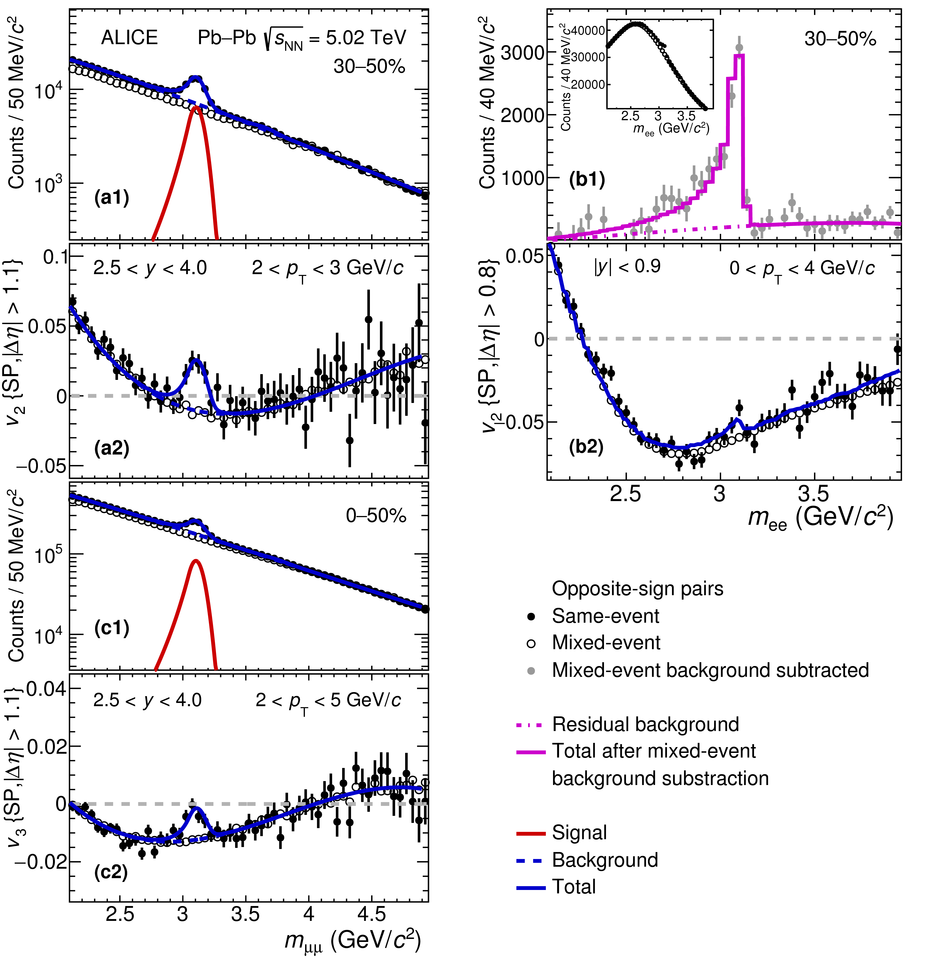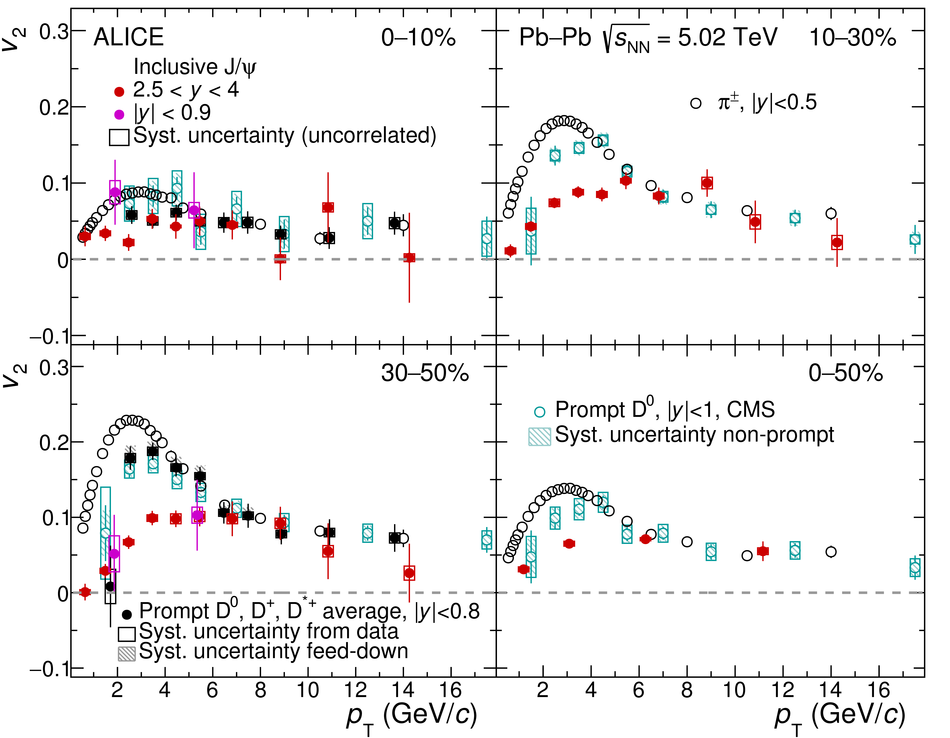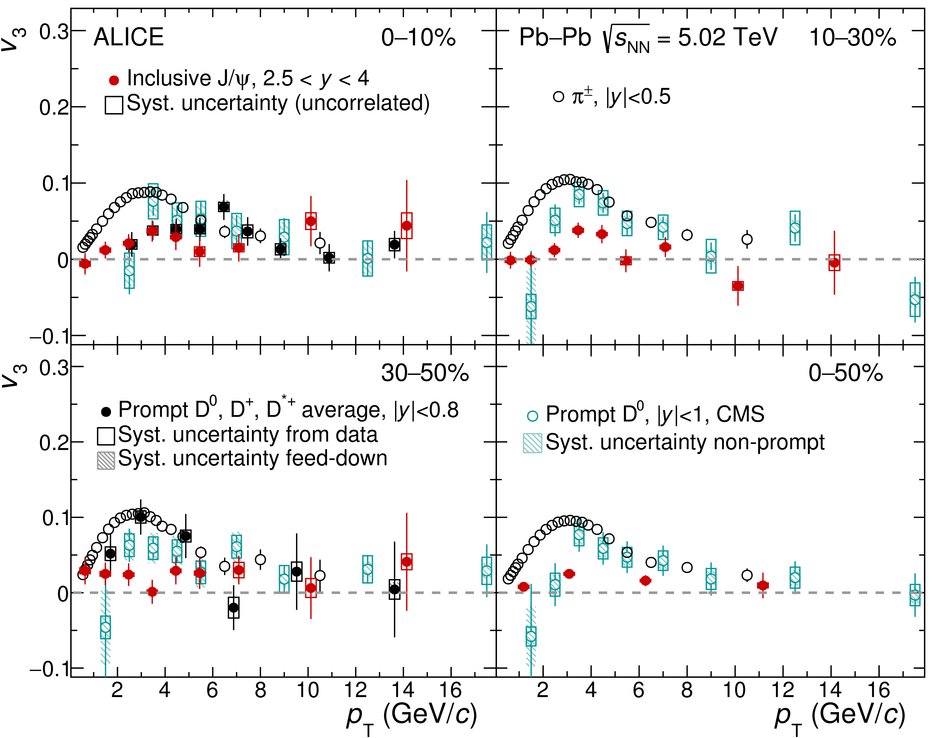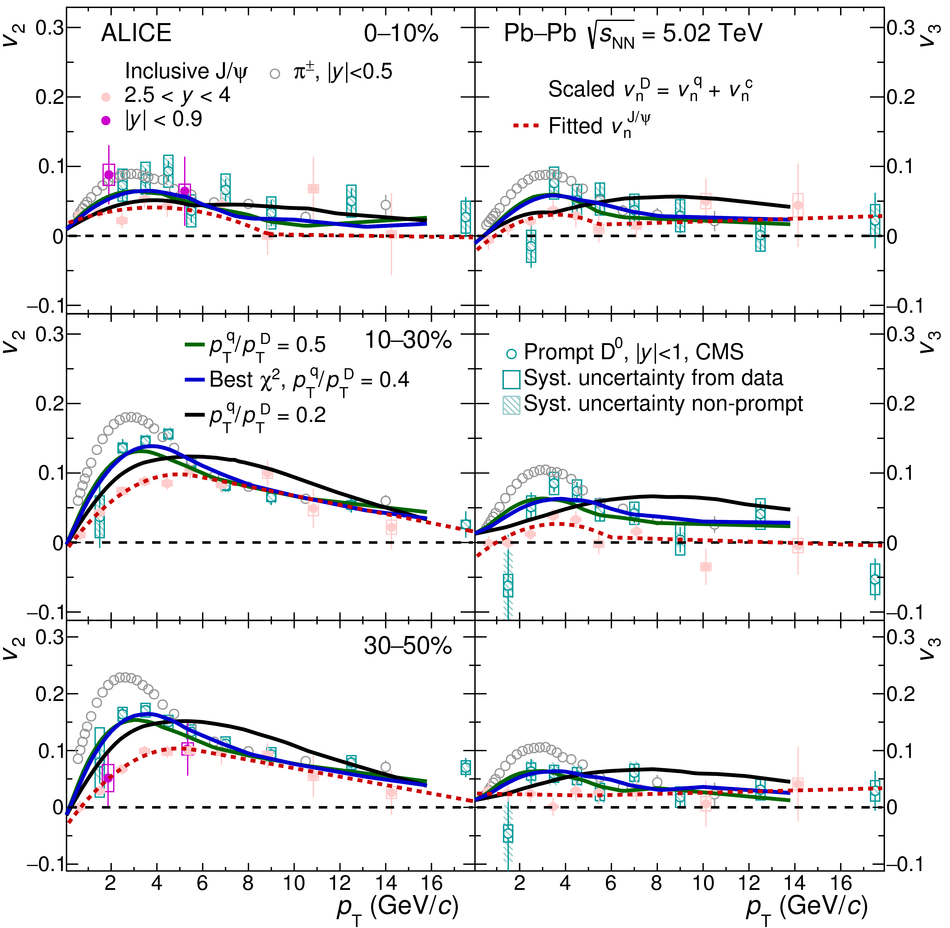# J/$ψ$ elliptic and triangular flow in Pb-Pb collisions at $\sqrt{s_{\rm NN}}$ = 5.02 TeV

The inclusive J/$\psi$ elliptic ($v_2$) and triangular ($v_3$) flow coefficients measured at forward rapidity (2.5 $<~y<~$ 4) and the $v_2$ measured at midrapidity ($|y|<~$ 0.9) in Pb-Pb collisions at $\sqrt{s_{\rm NN}}$ = 5.02 TeV using the ALICE detector at the LHC are reported. The entire Pb-Pb data sample collected during Run 2 is employed, amounting to an integrated luminosity of 750 $\mu$b$^{-1}$ at forward rapidity and 93 $\mu$b$^{-1}$ at midrapidity. The results are obtained using the scalar product method and are reported as a function of transverse momentum $p_{\rm T}$ and collision centrality. At midrapidity, the J/$\psi$ $v_2$ is in agreement with the forward rapidity measurement. The centrality averaged results indicate a positive J/$\psi$ $v_3$ with a significance of more than 5$\sigma$ at forward rapidity in the $p_{\rm T}$ range $2<~p_{\rm T}<~5$ GeV/$c$. The forward rapidity $v_2$, $v_3$, and $v_3$/$v_2$ results at low and intermediate $p_{\rm T}$ ($p_{\rm T} \lesssim 8$ GeV/$c$) exhibit a mass hierarchy when compared to pions and D mesons, while converging into a species-independent curve at higher $p_{\rm T}$. At low and intermediate $p_{\rm T}$, the results could be interpreted in terms of a later thermalization of charm quarks compared to light quarks, while at high $p_{\rm T}$, path-length dependent effects seem to dominate. The J/$\psi$ $v_2$ measurements are further compared to a microscopic transport model calculation. Using a simplified extension of the quark scaling approach involving both light and charm quark flow components, it is shown that the D-meson $v_{\rm n}$ measurements can be described based on those for charged pions and J/$\psi$ flow.

Submitted to: JHEP
HEP Data
e-Print: arXiv:2005.14518 | PDF | inSPIRE
CERN-EP-2020-094

Figures

## Figure 1

 Invariant mass distribution (top panels \textbf{a1}, \textbf{b1}) and $\elflow(\mll)$ (bottom panels \textbf{a2}, \textbf{b2}) for dimuons in the ranges $2 < \pt < 3$~\gevc (top left) and for dielectrons in $0 < \pt < 4$~\gevc (top right), for the 30--50\% centrality interval. Fit functions of the invariant mass distributions and $\elflow(\mmumu)$, as discussed in Sec.~\ref{section:analysis_details}, are also shown. Bottom panel, invariant mass (\textbf{c1}) and $\triflow(\mmumu)$ (\textbf{c2}) distributions for dimuons in the \pt range $2 < \pt < 5$~\gevc for the 0--50\% centrality interval. The $\flown(\mmumu)$ and $\elflow(\mee)$ distributions are plotted with the background flow obtained from the event-mixing procedure and the fit function, as discussed in the text. Only statistical uncertainties are shown.## Figure 2

 Inclusive \jpsi \elflow as function of \pt in different centrality intervals (0--10\%, 10--30\%, 30--50\% and 0--50\%) in \pbpb collisions at \fivenn. Both midrapidity and forward rapidity \jpsi \elflow measurements are shown. The results are compared with the \elflow coefficients at midrapidity for charged pions~ and prompt $D^{0}$ mesons~. The statistical and systematic uncertainties are shown as bars and boxes, respectively. The shaded cyan boxes represent the systematic uncertainties from the contribution of non-prompt $D^{0}$ mesons.## Figure 3

 Inclusive \jpsi \triflow at forward rapidity as function of \pt in different centrality intervals (0--10\%, 10--30\%, 30--50\% and 0--50\%) in \pbpb collisions at \fivenn. The results are compared to the \triflow coefficients at midrapidity for charged pions~ and prompt $D^{0}$ mesons~. The statistical and systematic uncertainties are shown as bars and boxes, respectively. The shaded bands represent the systematic uncertainties from the contribution of non-prompt $D^{0}$ mesons.## Figure 4

 Ratio of \triflow to \elflow of inclusive \jpsi (left panel) and $v_{3}/v_{2}^{3/2}$ (right panel) at forward rapidity as a function of \pt for the 0--50\% centrality interval in \pbpb collisions at \fivenn. The results are compared with the flow coefficients of charged pions~ and prompt $D^{0}$ mesons at midrapidity . The statistical and systematic uncertainties are shown as bars and boxes. The shaded bands represent the systematic uncertainties from the contribution of non-prompt $D^{0}$ mesons.## Figure 5

 Inclusive J/$\psi$ $v_{2}$ as function of $p_{\rm T}$ at forward rapidity for semi-central (20–40\%) Pb–Pb collisions at \fivenn. Calculations from a transport model are also shown.## Figure 6

 The inclusive J/$\psi$ $v_{2}$ and $v_{3}$ as function of the centrality of the collision, at forward rapidity, for the low-$p_{\rm T}$ range $0< p_{\rm T}< 5$ GeV/$c$ (left panel) and high-$p_{\rm T}$ range $5< p_{\rm T}< 20$ GeV/$c$ (right panel). The results are compared to the $v_{\rm n}$ coefficients of midrapidity $\pi^{\pm}$~ at low and high-$p_{\rm T}$ corresponding to $1.75< p_{\rm T}< 2$ GeV/$c$ and $6< p_{\rm T}< 7$ GeV/$c$, respectively. The ratio of midrapidity $\pi^{\pm}$ $v_{2}$ to inclusive J/$\psi$ $v_{2}$ is also shown.## Figure 7

 Elliptic (left panels) and triangular (right panels) flow of inclusive \jpsi , D-mesons and charged pions as a function of \pt for the centrality intervals 0--10\% (top), 10--30\% (middle) and 30--50\% (bottom). The continuous curves show the calculated D-meson flow based on different values of the \pt fraction carried by the light quark (see text). The red dashed curves show the fits to the \jpsi \flown using ad-hoc functions (see text).